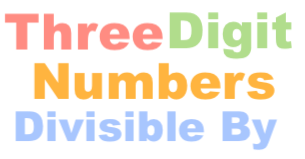Three digit numbers divisible by 13We will start off with a couple of definitions for clarification. Three digit numbers (3-digit numbers) are numbers that have three digits in them. They range from 100 to 999. Therefore, there are a total of 900 3-digit numbers.

Furthermore, a three digit number is divisible by 13 if you divide the three digit number by 13 and you get a whole number with no remainder.

Below you will find many questions and answers related to three digit numbers divisible by 13.

How many three digit numbers are divisible by 13?
Below is a list of all three digit numbers divisible by 13 in chronological order. There are 69 three digit numbers divisible by 13.

104, 117, 130, 143, 156, 169, 182, 195, 208, 221, 234, 247, 260, 273, 286, 299, 312, 325, 338, 351, 364, 377, 390, 403, 416, 429, 442, 455, 468, 481, 494, 507, 520, 533, 546, 559, 572, 585, 598, 611, 624, 637, 650, 663, 676, 689, 702, 715, 728, 741, 754, 767, 780, 793, 806, 819, 832, 845, 858, 871, 884, 897, 910, 923, 936, 949, 962, 975, 988

How many three digit numbers are NOT divisible by 13?
Like we wrote above, there are a total of 900 three digit numbers and 69 of them are divisible by 13. Furthermore, 900 - 69 = 831. Therefore, there are 831 three digit numbers that are not divisible by 13.

What is the sum of all three digit numbers divisible by 13?
We added up all the 3-digit numbers in our list above. The sum of all 3-digit numbers divisible by 13 is 37674.

What is the smallest three digit number divisible by 13?
The smallest or lowest 3-digit number divisible by 13 is the first number on the list above (first 3 digit number divisible by 13). As you can see, that number is 104.

What is the largest three digit number divisible by 13?
The largest or greatest 3-digit number divisible by 13 is the last number on the list above (last 3 digit number divisible by 13). As you can see, that number is 988.

How many even three digit numbers are divisible by 13?
Below is a list of all 3-digit EVEN numbers divisible by 13 in chronological order. There are 35 even three digit numbers divisible by 13.

104, 130, 156, 182, 208, 234, 260, 286, 312, 338, 364, 390, 416, 442, 468, 494, 520, 546, 572, 598, 624, 650, 676, 702, 728, 754, 780, 806, 832, 858, 884, 910, 936, 962, 988

How many odd three digit numbers are divisible by 13?
Below is a list of all 3-digit ODD numbers divisible by 13 in chronological order. There are 34 odd three digit numbers divisible by 13.

117, 143, 169, 195, 221, 247, 273, 299, 325, 351, 377, 403, 429, 455, 481, 507, 533, 559, 585, 611, 637, 663, 689, 715, 741, 767, 793, 819, 845, 871, 897, 923, 949, 975

Three Digit Numbers Divisible By Calculator
There you have it folks! Your are now a "Three digit numbers divisible by 13" expert. You can enter another number below for us to tackle!

Three digit numbers divisible by 14
Here is a similar problem we have explained and answered.